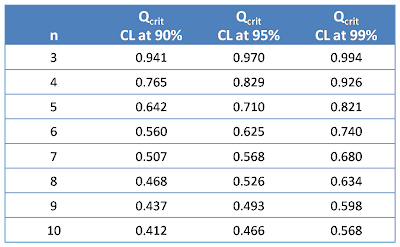## Monday, 29 June 2009

### Dealing with outliers in analytical method validation

ANALYTICAL TOPICS

Occasionally in validation studies, as in any analysis, outliers may be observed in data sets of results. This is where a value is present in the data set which differs considerably from the majority of the other results. For example, the following data set was obtained for a precision study:

25.4, 25.3, 27.5, 24.5, 24.7, 25.6

The value 27.5 is much higher than the one nearest to it at 25.6 and it is suspected that it may be an outlier. The mean and standard deviation calculated for the data set including and excluding the suspected outlier are presented in Table 1. The mean does not appear to be effected substantially by the suspected outlier but if the suspect value is included the %RSD does not comply with the acceptance criterion of %RSD ≤ 2% for precision. If it is excluded the precision complies with the acceptance criterion.

Table 1Statistical tests can be performed which will provide confidence in the characterisation of a data point as an outlier. However a decision still has to be made about whether to exclude the data point from the results. Some statisticians object to the rejection of any data from small size data sample, unless it is known that something went wrong during the measurement of that data. Rejection of data during validation studies must be very carefully considered, statistical evidence alone may not be enough to justify the rejection of data.

The most popular statistical test applied to detect suspect outliers in the results from chemical analysis is Dixon’s Q-test. One (and only one) observation from a small set of replicate observations (typically 3 to 10) can be examined. The test assumes a normal distribution of the data. The null hypothesis for this test is that there is no significant difference between the suspect value and the rest of the values, any differences must be attributed to random errors.

The test is applied as follows:

1. The values comprising the data set are arranged in ascending order:
X1, X2, X3, ........ Xn

e.g. 24.5, 24.7, 25.3, 25.4, 25.6, 27.5

2. The experimental Q-value is calculated, defined as the ratio of the difference between the suspect value and the nearest value to it, to the range of the data.

If the suspected outlier is a low value:If the suspected outlier is a high value:e.g. Qexp = (27.5 – 25.6)/(27.5 – 24.5) = 0.633

3. The value of Qexp is compared to a critical Q-value (Qcrit). Refer to Table 2 for critical values of Q for Dixon’s test, from work by Rorabacher. The value of Qcrit corresponding to the confidence level required for the test is selected, usually 95%.

e.g. Qcrit = 0.625 (95% confidence level)

4. If Qexp > Qcrit, then the suspect value can be characterised as an outlier.

e.g. Qexp > Qcrit, therefore data point 27.5 can be characterised as an outlier.

Table 2The problems associated with the Q-test are that it may be misleading if more than one outlier is present, and also if an outlier is detected, a decision then has to be made about whether to exclude the data point from further statistical calculations. Methods which are more robust than the Q-test, such as the Huber method (described in the AMC technical brief, no. 6), are becoming increasingly favoured for treatment of outliers since they consider all data present in the set, and not only three data points as in the Q-test. Robust statistics utilise approaches such as the use of the median and median absolute difference to estimate the mean and standard deviation respectively. In this way the outlying data has no effect and the does not have to be rejected. Any approach used to deal with outliers will have to be justified fully in the validation report.

References:
1. D. B. Rorabacher, Anal. Chem, 63, 139-146, 1991, ‘A Statistical treatment for rejection of deviant values: critical values of Dixon's "Q" parameter and related subrange ratios at the 95% confidence level’.
2. Analytical Methods Committee, AMC Technical Brief, No. 6, 2007, ‘Robust statistics: a method of coping with outliers’ (available on RSC website, http://www.rsc.org/).

This blog post is an excerpt from 'Validation of Analytical Methods for Pharmaceutical Analysis' by Oona McPolin, available to purchase through the MTS website.